# NCERT Exemplar Problems and their Solutions

Reviewed By:
Krishna Kant Majee
M.Sc., B.Ed.

### Chapter 5-Periodic Classification of Elements-Long Answers

Q.42. An element is placed in 2nd group and 3rd period of the periodic table burns in presence of oxygen to form a basic oxide.
(a) Identify the element.
(b) Write the electronic configuration.
(c) Write a balanced equation when it burns in the presence of air.
(d) Write a balanced equation when this oxide is dissolved in water
(e) Draw the electron dot structure for the formation of this oxide.
(a) Given element is placed in group 2 so it must be alkaline earth metal. The alkaline earth metal in period 3 is magnesium (Mg).
(b) The atomic number of Mg is 12, and electronic configuration is :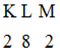(c) Magnesium burns in the presence of air to form magnesium oxide (MgO). It is a basic oxide.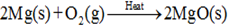(d) Basic oxide of magnesium; MgO is dissolved in water to form magnesium hydroxide.
$2MgO (s) + 2H2O (l) → 2Mg(OH)_{2} (aq)$
(e) Magnesium oxide is an ionic compound in which Mg exists as $Mg^{+2}$ ion and oxygen as $O^{-2}$ ion. The electron dot structure for the formation of magnesium oxide is given below.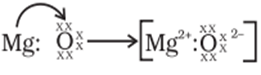Q.43. An element X (atomic number 17) reacts with an element Y (atomic number 20) to form a divalent halide.
(a) Where in the periodic table are elements X and Y placed?
(b) Classify X and Y as metal (s), non-metal (s) or metalloid (s).
(c) What will be the nature of oxide of element Y? Identify the nature of bonding in the compound formed.
(d) Draw the electron dot structure of the divalent halide.
(a) The atomic number of element X is 17 and electronic configuration is 2, 8, 7. There are 7 valence electrons so it must be placed in group 17 and period 3. It is chlorine. The atomic number of Y is 20 and electronic configuration is 2, 8, 8, 2. So there are 2 valence electrons and it must be a part of group 2 and 4th period as there are four shells. The element Y is calcium.
(b) Chlorine (element X) has 7 valence electrons hence it requires one electron to get the octet configuration. So it has tendency to accept one electron and form anion. Therefore it is a non-metal. Element Y has two valence electrons which can easily lose to get the stable electronic configuration of the nearest inert gas. Hence it is a metal
(c) Calcium is a metal and it will form basic oxides like calcium oxide (CaO) must be basic in nature. Here calcium is metal and oxygen is non-metal therefore the bonding between metals and non-metals must be ionic in nature.
(d) The chemical formula of divalent halide of calcium and chlorine would be $CaCl_{2}$ (calcium chloride). Electron dot structure for the formation of calcium chloride is given below;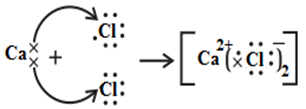Q.44. Atomic number of a few elements are given below
10, 20, 7, 14
(a) Identify the elements.
(b) Identify the group number of these elements in the periodic table.
(c) Identify the periods of these elements in the periodic table.
(d) What would be the electronic configuration for each of these elements?
(e) Determine the valency of these elements.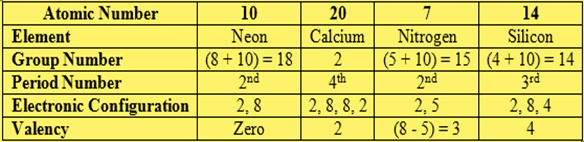Q.45. Complete the following crossword puzzle (Figure).
Across
(1) An element with atomic number 12.
(3) Metal used in making cans and member of group 14.
(4) A lustrous non-metal which has 7 electrons in its outermost shell.
Down
(2) Highly reactive and soft metal which imparts yellow colour when subjected to flame and is kept in kerosene.
(5) The first element of second period.
(6) An element which is used in making florescent bulbs and is second member of group 18 in the modern periodic table.
(7) A radioactive element which is the last member of halogen family.
(8) Metal which is an important constituent of steel and forms rust when exposed to moist air.
(9) The first metalloid in modern periodic table whose fibres are used in making bullet-proof vests.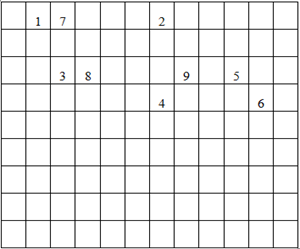Across
(1) Magnesium.
(3) Tin.
(4) Iodine.
Down
(2) Sodium.
(5) Lithium.
(6) Neon.
(7) Astatine.
(8) Iron.
(9) Boron.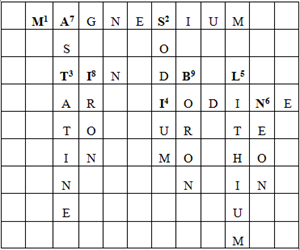Q.46. (a) In this ladder (Figure) symbols of elements are jumbled up. Rearrange these symbols of elements in the increasing order of their atomic numbers in the periodic table.
(b) Arrange them in the order of their group also.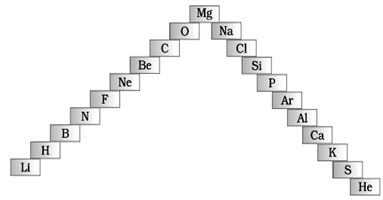(a) The symbols of elements in increasing order of their atomic numbers are:
H, He, Li, Be, B, C, N, O, F, Ne, Na, Mg, Al, Si, P, S, Cl, Ar, K, Ca.
(b) The symbols of elements in increasing order of their group numbers are as follows.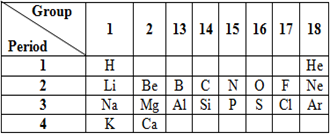Q.47. Mendeleev predicted the existence of certain elements not known at that time and named two of them as Eka-silicon and Eka-aluminium.
(a) Name the elements which have taken the place of these elements.
(b) Mention the group and the period of these elements in the modern periodic table.
(c) Classify these elements as metals, non-metals or metalloids.
(d) How many valence electrons are present in each one of them?
(a) Eka-silicon was later identified as Germanium (Ge) and Eka- aluminium was taken as Gallium (Ga).
(b)
• Germanium (Ge)- Group 14, period 4
• Gallium (Ga)- Group 13, period 4
(c) Gallium(Ga) is a metal while Germanium (Ge) is a metalloid.
(d) Gallium is placed in 13th group so there must be 13 – 10 = 3 valence electrons. Similarly germanium is placed in group 14 so there must be 14 – 10 = 4 valence electrons.
Q.48.
(a) Electropositive nature of the element (s) increases down the group and decreases across the period.
(b) Electronegativity of the element decreases down the group and increases across the period.
(c) Atomic size increases down the group and decreases across a period (left to right).
(d) Metallic character increases down the group and decreases across a period.
On the basis of the above trends of the periodic table, answer the following about the elements with atomic numbers 3 to 9.
(a) Name the most electropositive element among them.
(b) Name the most electronegative element.
(c) Name of the element with smallest atomic size.
(d) Name the element which is a metalloid.
(e) Name the element which shows maximum valency.
Elements from atomic number 3 to 9 are listed below:

 AtomicNumber Name Symbol 3 Lithium Li 4 Beryllium Be 5 Boron B 6 Carbon C 7 Nitrogen N 8 Oxygen O 9 Fluorine F

(a) Most electropositive element-Lithium (Li)
(b) Most electronegative element- Fluorine (F)
(c) Element with smallest atomic size- Fluorine (F)
(d) Metalloid element- Boron (B).
(e) Element with maximum valency- Carbon
Q.49. An element X which is a yellow solid at room temperature shows catenation and allotropy. X forms two oxides which are also formed during the thermal decomposition of ferrous sulphate crystals and are the major air pollutants.
(a) Identify the element X.
(b) Write the electronic configuration of X.
(c) Write the balanced chemical equation for the thermal decomposition of ferrous sulphate crystals?
(d) What would be the nature (acidic/basic) of oxides formed?
(e) Locate the position of the element in the modern periodic table.
(a) The yellow solid element which shows catenation and allotropy is sulphur (S).
(b) The atomic number of sulphur is 16 with electronic configuration as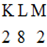(c) Thermal decomposition of ferrous sulphate $(FeSO_{4})$ forms ferric oxide (Fe2O3)with sulphur dioxide $(SO_{2})$ and sulphur trioxide $(SO_{3}).$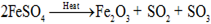(d) Both oxides of sulphur are acidic in nature as sulphur is a non-metal. Both $SO_{2}$ and $SO_{3}$ dissolve in water to form the corresponding acids.
$SO_{2} + H_{2}O → H_{2}SO_{3}$
$SO_{3} + H_{2}O → H_{2}SO_{4}$
(e) The electronic configuration of sulphur is 2, 8, 6 with six valence electrons. So it must be placed in 10 + 6 = 16th group of periodic table and period 3 as there are 3 shells in sulphur atom.
Q.50. An element X of group-15 exists as diatomic molecule and combines with hydrogen at 773 K in presence of the catalyst to form a compound, ammonia which has a characteristic pungent smell.
(a) Identify the element X. How many valence electrons does it have?
(b) Draw the electron dot structure of the diatomic molecule of X. What type of bond is formed in it?
(c) Draw the electron dot structure for ammonia and what type of bond is formed in it?
(a) Element X which is placed in group 15 must be nitrogen (N). As it exists as diatomic molecule (N2) and combine with hydrogen to ammonia. The electronic configuration of nitrogen is 2, 5 hence it has 5 valence electrons.
(b) Nitrogen has 5 valence electrons, so it requires three more electrons to get the octet configuration. Hence it tends to share its three electrons and form nitrogen molecule in which both nitrogen atoms are bonded via triple covalent bond. The electron dot structure of nitrogen molecule is given below.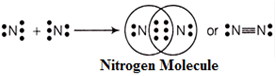(c) The chemical formula of ammonia is $NH_{3}$. Here center nitrogen atom is bonded with three hydrogen atoms through three single covalent bonds. The electron dot structure for ammonia is as follows.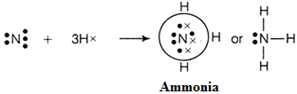Q.51. Which group of elements could be placed in Mendeleev’s periodic table without disturbing the original order? Give reason.